The GLIMMIX Procedure
 Generalized Linear Models Theory

A generalized linear model consists of the following:

• a linear predictor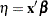• a monotonic mapping between the mean of the data and the linear predictor

• a response distribution in the exponential family of distributions

A density or mass function in this family can be written as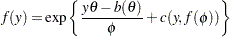for some functions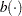and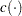. The parameter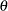is called the natural (canonical) parameter. The parameter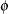is a scale parameter, and it is not present in all exponential family distributions. See Table 38.15 for a list of distributions for which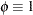. In the case where observations are weighted, the scale parameter is replaced with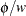in the preceding density (or mass function), whereis the weight associated with the observation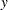.

The mean and variance of the data are related to the components of the density,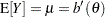,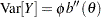, where primes denote first and second derivatives. If you expressas a function of, the relationship is known as the natural link or the canonical link function. In other words, modeling data with a canonical link assumes that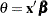; the effect contributions are additive on the canonical scale. The second derivative of, expressed as a function of, is the variance function of the generalized linear model,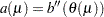. Note that because of this relationship, the distribution determines the variance function and the canonical link function. You cannot, however, proceed in the opposite direction. If you provide a user-specified variance function, the GLIMMIX procedure assumes that only the first two moments of the response distribution are known. The full distribution of the data is then unknown and maximum likelihood estimation is not possible. Instead, the GLIMMIX procedure then estimates parameters by quasi-likelihood.Previous Page | Next Page | Top of Page